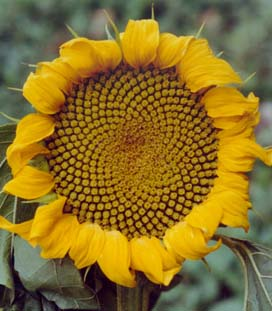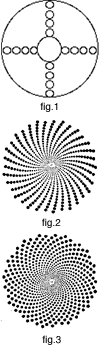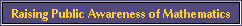Flower Patterns and Fibonacci NumbersSunflower (photo by Yves Couder)

Why is it that the number of petals in a flower is often one of the following numbers: 3, 5, 8, 13, 21, 34 or 55? For example, the lily has three petals, buttercups have five of them, the chicory has 21 of them, the daisy has often 34 or 55 petals, etc. Furthermore, when one observes the heads of sunflowers, one notices two series of curves, one winding in one sense and one in another; the number of spirals not being the same in each sense. Why is the number of spirals in general either 21 and 34, either 34 and 55, either 55 and 89, or 89 and 144? The same for pinecones : why do they have either 8 spirals from one side and 13 from the other, or either 5 spirals from one side and 8 from the other? Finally, why is the number of diagonals of a pineapple also 8 in one direction and 13 in the other?

Are these numbers the product of chance? No! They all belong to the Fibonacci sequence: 1, 2, 3, 5, 8, 13, 21, 34, 55, 89, 144, etc. (where each number is obtained from the sum of the two preceding). A more abstract way of putting it is that the Fibonacci numbers fn are given by the formula f1 = 1, f2 = 2, f3 = 3, f4 = 5 and generally f n+2 = fn+1 + fn . For a long time, it had been noticed that these numbers were important in nature, but only relatively recently that one understands why. It is a question of efficiency during the growth process of plants (see below).

The explanation is linked to another famous number, the golden mean, itself intimately linked to the spiral form of certain types of shell. Let's mention also that in the case of the sunflower, the pineapple and of the pinecone, the correspondence with the Fibonacci numbers is very exact, while in the case of the number of flower petals, it is only verified on average (and in certain cases, the number is doubled since the petals are arranged on two levels).

Let's underline also that although Fibonacci historically introduced these numbers in 1202 in attempting to model the growth of populations of rabbits, this does not at all correspond to reality! On the contrary, as we have just seen, his numbers play really a fundamental role in the context of the growth of plants

THE EFFECTIVENESS OF THE GOLDEN MEAN

The explanation which follows is very succinct. For a much more detailed explanation, with very interesting animations, see the web site in the reference.

In many cases, the head of a flower is made up of small seeds which are produced at the centre, and then migrate towards the outside to fill eventually all the space (as for the sunflower but on a much smaller level). Each new seed appears at a certain angle in relation to the preceeding one. For example, if the angle is 90 degrees, that is 1/4 of a turn, the result after several generations is that represented by figure 1.Of course, this is not the most efficient way of filling space. In fact, if the angle between the appearance of each seed is a portion of a turn which corresponds to a simple fraction, 1/3, 1/4, 3/4, 2/5, 3/7, etc (that is a simple rational number), one always obtains a series of straight lines. If one wants to avoid this rectilinear pattern, it is necessary to choose a portion of the circle which is an irrational number (or a nonsimple fraction). If this latter is well approximated by a simple fraction, one obtains a series of curved lines (spiral arms) which even then do not fill out the space perfectly (figure 2).

In order to optimize the filling, it is necessary to choose the most irrational number there is, that is to say, the one the least well approximated by a fraction. This number is exactly the golden mean. The corresponding angle, the golden angle, is 137.5 degrees. (It is obtained by multiplying the non-whole part of the golden mean by 360 degrees and, since one obtains an angle greater than 180 degrees, by taking its complement). With this angle, one obtains the optimal filling, that is, the same spacing between all the seeds (figure 3).

This angle has to be chosen very precisely: variations of 1/10 of a degree destroy completely the optimization. (In fig 2, the angle is 137.6 degrees!) When the angle is exactly the golden mean, and only this one, two families of spirals (one in each direction) are then visible: their numbers correspond to the numerator and denominator of one of the fractions which approximates the golden mean : 2/3, 3/5, 5/8, 8/13, 13/21, etc.

These numbers are precisely those of the Fibonacci sequence (the bigger the numbers, the better the approximation) and the choice of the fraction depends on the time laps between the appearance of each of the seeds at the center of the flower.

This is why the number of spirals in the centers of sunflowers, and in the centers of flowers in general, correspond to a Fibonacci number. Moreover, generally the petals of flowers are formed at the extremity of one of the families of spiral. This then is also why the number of petals corresponds on average to a Fibonacci number.

REFERENCES:

1. Ron Knot's excellent internet site at the University of Surrey on this and related topics.

2. S. Douady et Y. Couder, La physique des spirales végétales, La Recherche, janvier 1993, p. 26 (In French).© Mathematics and Knots, U.C.N.W.,Bangor, 1996 - 2002
This material may be used freely for educational, artistic and scientific purposes, but may not be used for commercial purposes, for profit or in texts without the permission of the publishers.